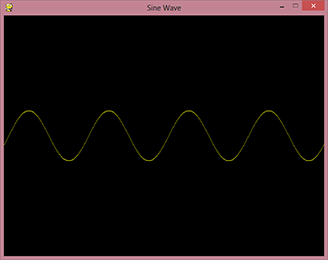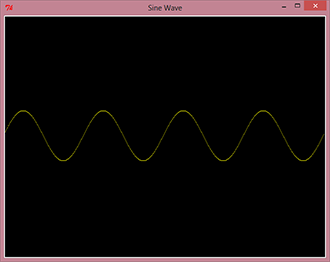Heya, Thanks for visiting!

# Animated Sine Wave two-ways with pygame and tkinter

• canvas
• pygame
• tkinter
• python

I decided to post up this simple script that generates an animated sine wave that scrolls horizontally. I built both versions because I need to use a canvas and some bare-bone pixel manipulation for a future project and want to find the best solution.

Update (2013-12-11): I decided to use JavaScript and the HTML5 `<canvas>` for the project I ended up making. Go check out the VGA Simulator

The first one is made with pygame, which runs smooth and is straight forward efficient. The second version made with tkinter is slow and laggy. I even had to batch edit pixels which does not help much. Both of them function exactly the same but the tkinter version is just not as fast.

All you need is Python 2.7 and pygame installed. If you are on Windows, you might consider getting a nice binary from here. This is also a great project to get started with pygame.``````import pygame
import time
import math

# Some config width height settings
canvas_width = 640
canvas_height = 480

# Just define some colors we can use
color = pygame.Color(255, 255, 0, 0)
background_color = pygame.Color(0, 0, 0, 0)

pygame.init()
# Set the window title
pygame.display.set_caption("Sine Wave")

# Make a screen to see
screen = pygame.display.set_mode((canvas_width, canvas_height))
screen.fill(background_color)

# Make a surface to draw on
surface = pygame.Surface((canvas_width, canvas_height))
surface.fill(background_color)

# Simple main loop
running = True
while running:
for event in pygame.event.get():
if event.type == pygame.QUIT:
running = False

# Redraw the background
surface.fill(background_color)

# Update sine wave
frequency = 4
amplitude = 50 # in px
speed = 1
for x in range(0, canvas_width):
y = int((canvas_height/2) + amplitude*math.sin(frequency*((float(x)/canvas_width)*(2*math.pi) + (speed*time.time()))))
surface.set_at((x, y), color)

# Put the surface we draw on, onto the screen
screen.blit(surface, (0, 0))

# Show it.
pygame.display.flip()``````NOTE: For python 3.x: change the import statement at the top to `from tkinter import ...` ("..." is the rest of that line) - The package changes to lowercase `T` in 3.x. The uppercase Tkinter package is for python 2.x

``````from Tkinter import Tk, Canvas, PhotoImage, mainloop
import math
import time

# Used to store debug file
#import os
#BASE_DIR = os.path.realpath(os.path.dirname(__file__))

# Some config width height settings
canvas_width = 640
canvas_height = 480

# Create a window
window = Tk()
# Set the window title
window.wm_title("Sine Wave")

# Put a canvas on the window
canvas = Canvas(window, width=canvas_width, height=canvas_height, bg="#000000")
canvas.pack()

# Create a image, this acts as the canvas
img = PhotoImage(width=canvas_width, height=canvas_height)

# Put the image on the canvas
canvas.create_image((canvas_width/2, canvas_height/2), image=img, state="normal")

def sine_wave_anim():
# Update sine wave
frequency = 4
amplitude = 50 # in px
speed = 1

# We create a blank area for what where we are going to draw
color_table = [["#000000" for x in range(0, canvas_width)] for y in range(0, amplitude*2)]

# And draw on that area
for x in range(0, canvas_width):
y = int(amplitude + amplitude*math.sin(frequency*((float(x)/canvas_width)*(2*math.pi) + (speed*time.time()))))
color_table[y][x] = "#ffff00"

# Don't individually put pixels as tkinter sucks at this
#img.put("#ffff00", (x, y))

# Then batch put it on the canvas
# tkinter is extremely inefficient doing it one by one
img.put(''.join("{" + (" ".join(str(color) for color in row)) + "} " for row in color_table), (0, int(canvas_height/2 - amplitude)))

# Debug the color_table
#with open(os.path.join(BASE_DIR, 'output.txt'), "w+") as text_file:
#	text_file.write(''.join("{" + (" ".join(str(color) for color in row)) + "} " for row in color_table))

# Continue the animation as fast as possible. A value of 0 (milliseconds), blocks everything.
window.after(1, sine_wave_anim)

# Start off the anim
sine_wave_anim()
mainloop()``````